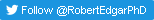﻿ Expected errors predicted by Phred (Q) scores11-Aug-2018 New paper describes octave plots for visualizing alpha diversity.

12-Jun-2018 New paper shows that one in five taxonomy annotations in SILVA and Greengenes are wrong.

18-Apr-2018 New paper shows that taxonomy prediction accuracy is <50% for V4 sequences.

05-Oct-2017 PeerJ paper shows low accuracy of closed- and open-ref. QIIME OTUs.

22-Sep-2017 New paper shows 97% threshold is wrong, OTUs should be 99% full-length 16S, 100% for V4.

24-Nov-2016Expected errors predicted by Phred (Q) scores

Setting an e.e. threshold for long reads

Phred scores
Next-generation reads specify a Phred score for each base, also known as a Quality or Q score. The Q score is an integer, typically in the range 2 to 40. Q indicates the probability that the base call is incorrect (P_e). For example, Q=2 means that the error probability is 63%, so the machine is reporting that the base is more likely to be wrong than right, while Q=20 corresponds to an error probability of 1%.

Expected number of errors
Assume that the Phred scores are good, in other words assume that the machine makes good estimates of the error probabilities. Now we can measure the overall quality of a read by calculating the expected number of errors in the read. The expected value of a quantity under a probability distribution is a technical term in statistics, so let me explain what that means in this case.

Take the error probabilities according to the Q scores in a given read, and imagine a very large number of reads containing errors that occur with those probabilities. For example, if the first base in the read has Q=20, then 1% of the reads in our collection will have errors in that position. Some of the reads will have no errors, some will have one error, and so on. The Q scores can't tell us exactly how many errors there are in a given read. However, the Q scores can tell us what the average number of errors would be in that large collection, and this is the definition of the expected number of errors. Because we're taking an average, the value is real rather than integer, and can be less than one.

Calculating the expected number of errors and most probable number of errors
It turns out that it is easy to calculate the expected number of errors: it is the sum of the error probabilities. The most probable number of errors (E*) is also easy to calculate. First calculate E = expected errors = sum P_e. Then round down to the nearest integer, and this is the most probable number of errors. In symbols: E* = floor(E). For example, if E < 1, then the most probable number of errors is zero. Proofs of these results are given in Edgar & Flybjerg (2014).

Quality filtering by expected errors
Small E means high quality, large E means low quality. We can therefore filter low quality reads by setting a maximum number of expected errors (E_max) and discarding reads with E > E_max. This is done by running the fastq_filter command.

Choosing the maximum expected error threshold
A natural choice is E_max = 1, because the most probable number of errors of a filtered read is zero. Though of course, we expect some of them to have one or more errors (this can't be entirely avoided, however stringent the filter, because Q scores are probabilities, not certainties). If you want to filter more stringently, you might choose something like E_max = 0.5 or E_max = 0.25.